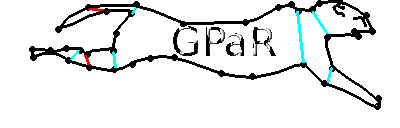Tutorial

Configuration

Environment managment

Graph managment

Rules managment

Execution managment

# GPaR 1.0x

GPaR is a parallel graph rewriting software implemented in C++ with a graphical user interface. This freeware tool has been developed by Grenoble University.GPaR main goal is to rewrite a graph denoted g into a graph g' by using, simultaneously, rewriting rules.
GPaR deals with overlapping matches and thus can be used for a large variety of rewriting problems including cellular automata or L-systems.
To tackle this problem of overlapping matches, an extended definition of graph is used. Usually, a graph is defined by an order pair (V,E) where V is the set of vertices and E a set of edges. Here, two types of vertices are identified: V=N ∪ P where N is a set of attributed nodes and P the set of attributed ports. Ports have a central role in ensuring the effective connection between the remaining parts of g in g' and the new parts. The methods used in GPaR are based on the theoretical results of "Parallel graph rewriting with overlapping rules" of Rachid Echahed and Aude Maignan ( CoRR, abs/1701.06790, 2017).

Formally, we define a general structure called pregraph :
A pregraph H is a tuple H= (NH, PH, PNH, PPH, AttH, attH) such that:
• NH is a finite set of nodes and PH is a finite set of ports,
• PNH is a relation PNH ⊆ PH × NH,
• PPH is a symmetric binary relation on ports, PPH ⊆PH × PH,
• AttH is a structure of attributes,
• attH is a function attH: PH ⊎ NH → 2AttH
• such that ∀ x ∈ NH ⊎ PH, card(attH(x)) is finite.
In this context, a graph, g, corresponds to a pregraph g=(N, P, PN, PP, Att, att) such that:
• PN is a relation ⊆ P × N which associates at most one node to every port.
• PP is a symmetric binary relation which associates at most one port to another port.
For a system of rewriting rules R = {li → ri, i = 1 . . . n}, the left-hand sides li and the right-hand sides ri must be graphs. GPaR rewrites the graph g into the graph g' by firing, simultaneously, the rules of R whose left-hand sides match subgraphs of g. Two parallel rewrite relations have been implemented in GPaR:
• full parallel rewrite relation: g is rewritten in g' using all the possible matches (including eventual permutations of the same set of nodes and ports).
• parallel rewrite relation up to automorphisms: If a set of matches is obtained from one rewrite rule on a same set of nodes and ports then only one match of this set is used during the rewriting process. Confluence results can be found in the paper cited above.
Once the initial graph g, the rules and the rewrite relation are defined, GPaR proceeds sequentially to the following tasks :
1. It selects all the subgraphs of g whose elements match one of the pattern li,
2. If the parallel rewrite relation "is up to automorphism" then it reduces the set of matches to the set of matches up to automorphism;
3. It replaces all the matches of all the li by a variant of ri. The states of nodes and port are computed according to the rules. A pregraph has been created.
4. It merges ports and edges accordingly to the rewriting modulo approach.
GPaR is a standalone software whose graphical user interface is inherited from the Qt library. It is based on Boost Graph Library to match subgraphs thanks to the BGL vf2 subgraph isomorphism algorithm routine (see Herb Sutter and Andrei Alexandrescu Boost c++ libraries).
It also uses a mathematical expression library based on Fast c++ math expression parser and evaluator to manage the rewriting rule definitions and graph state definition.
GPar is composed of several windows which are